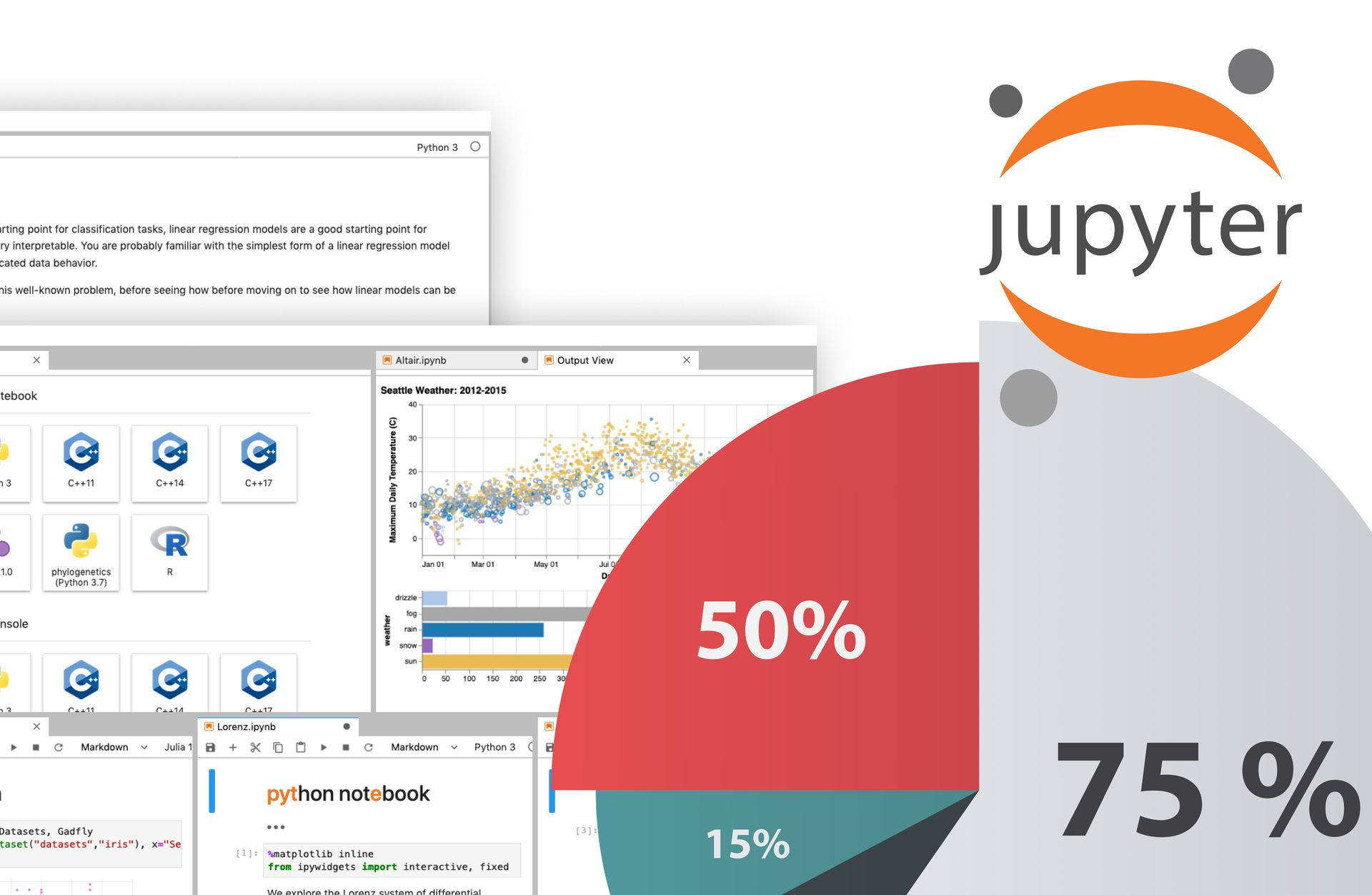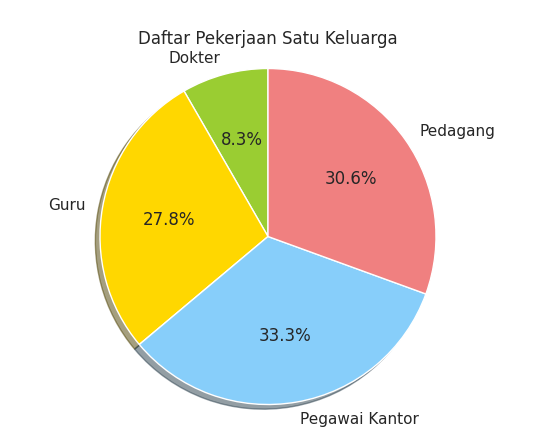Visualizing Data to Pie Chart Using Jupyter Notebook
How to visualize simple data to pie chart using Jupyter NotebookBefore we talk about how to realize or visualize data in Jupyter Notebook,  you should to understand what data visualization means first. As we know, the meaning of visualization is realizing or presenting something in the form of writing or numbers which we can call complex data in the form of images or graphics so that it is easier for users to understand and digest the data. Usually the data that has been processed will be displayed in the form of bar charts, line charts and pie charts etc.### Why Data Must be VisualizeSource : Freepik

Data analysts often face problems reading and understanding data if the data is not visualized. Without visualization, data analysts have to read each number individually and try to understand any possible patterns and trends. This can be a time consuming and challenging task, especially if the data is very large or complex. Without visualization, data analysts also run the risk of misinterpreting information or drawing wrong conclusions.

Data visualization helps data analysts to understand information more quickly and easily. By looking at data in the form of graphs or charts, data analysts can easily find patterns and trends that might not be apparent by looking at the numbers alone. Data visualization also helps data analysts to make more precise conclusions and facilitates communication of analysis results to colleagues or stakeholders.

In other words, visualizing data is a very important tool for data analysts to understand and present data more effectively. Without visualization, the data analyst's job would be more difficult and require more time and effort to achieve the same results. Therefore, data visualization must be an integral part of the data analysis process.

### Why Jupyter Notebook

Jupyter Notebook is one of those useful tools for visualizing data and performing data analysis. This application allows data analysts to write, execute, and present analysis results in one place. Jupyter Notebook has an intuitive interface and enables data analysts to easily take notes, format text, add images and data visualizations, and share analysis results.

By using Jupyter Notebook, data analysts can easily visualize data and perform data analysis interactively. This streamlines the analysis process and ensures that data analysts fully understand each step taken. Jupyter Notebook also enables data analysts to work collaboratively with colleagues or friends, take notes, and better present analysis results.

Overall, Jupyter Notebook is a very useful tool for data analysts who want to visualize data and perform data analysis effectively. With Jupyter Notebook, data analysts can easily visualize data, perform analysis, and present analytical results well.

Ok, after we understand about the Data Visualize and why it is important, now is the time for us to try to create a data visualization that is easy and you can apply it using Jupyter Notebook.

### Data Visualization Using Jupyter Notebook (Pie Chart)

To make a pie chart using Jupyter Notebook, there are several steps we have to do :

#### 1. Install Jupyter Notebook

Jupyter notebook is based on python. So, before installing jupyter notebook you need to install python and other libraries first. After that you can use Jupyter Notebook to create data, you have to install Jupyter Notebook first. Here's how to install Jupyter Notebook on Linux, Windows, and Mac operating systems:

Linux:

2. Install pip by typing the command "sudo apt-get install python-pip"
3. Install Jupyter Notebook by typing the command "pip install jupyter"

Windows:

1. Open Command Prompt (cmd) as administrator
2. Install pip (Package Installer for Python) by typing the command "python -m ensurepip --upgrade"
3. Install Jupyter Notebook by typing the command "pip install jupyter"

Mac:

1. Open Terminal
2. Install pip by typing the command "sudo easy_install pip"
3. Install Jupyter Notebook by typing the command "pip install jupyter"

Wait until the installation process is complete and then, you can start Jupyter Notebook by typing the command "jupyter notebook" in Terminal (Linux or Mac) or Command Prompt (Windows). Then, you will be redirected to the web browser to open Jupyter Notebook.

#### 2. Install Library

To create a pie chart using Jupyter Notebook, we need the Python Library Matplotlib. Here's the step-by-step process to install Matplotlib on Linux, Windows, and Mac operating systems:

1. Linux:
b. Install pip by typing the command "sudo apt-get install python-pip"
c. Install Matplotlib by typing the command "pip install matplotlib"

2. Windows:
a. Open Command Prompt (cmd) as administrator
b. Install pip (Package Installer for Python) by typing the command "python -m ensurepip --upgrade"
c. Install Matplotlib by typing the command "pip install matplotlib"

3. Mac:
a. Open Terminal
b. Install pip by typing the command "sudo easy_install pip"
c. Install Matplotlib by typing the command "pip install matplotlib"

Get more important information :
How to Create Graph View in Odoo How to Adda Calendar View at Menus in Odoo Relational Fields in Odoo

Wait until the installation process is complete and then, you can check if Matplotlib has been installed correctly by running the command "import matplotlib" in Terminal (Linux or Mac) or Command Prompt (Windows). If there are no errors, it means that Matplotlib has been installed correctly and is ready to use.

#### 3. Create Pie Chart

A pie chart is a commonly used graph that represents data in the form of a divided circle, similar to a sliced pie. The following example shows data on the occupations of family members. Here's the example code:

`import matplotlib.pypilot as pltlabels = ['Dokter', 'Guru', Pegawai Kantor', 'Pedagang']quantity = [3,10,12,11]colors = ['yellowgreen','gold','lightskyblue','lightcoral']plt.title ('Daftar Pekerjaan Satu Keluarga')plt.pie(quantity, labels=labels,colors=colors, autopct='%1.1f%%', shadow=True, startangle=90)plt.axis('equal')plt.show()`

Description:

1. Labels → Labels for each data
2. Quantity → Quantity of each label
3. Colors → Colors for each label
4. plt.title → Title of the graph
5. plt.pie → Function to combine labels, quantity, and colors. The shadow is used to give a shadow effect, startangle=90 is used to make the first value equal to 90 degrees.
6. plt.axis → Provides aspect ratio for each value
7. plt.show → Displays the graph#### 4. The Result#### Conclusion :

Visualizing data with Jupyter Notebook makes it easy to display and understand data visually. Using programming languages like Python and libraries like Matplotlib, it is easy to process and create various types of plots and graphs. Its ease of access, integration with code, and task sharing make Jupyter Notebook a popular choice in the data analysis process.

If you're interested in learning Jupyter Notebook, reach out to Panemu for guidance and training. If you have any confusion regarding the explanations in this article, don't hesitate to ask.Bastomi 15 February, 2023